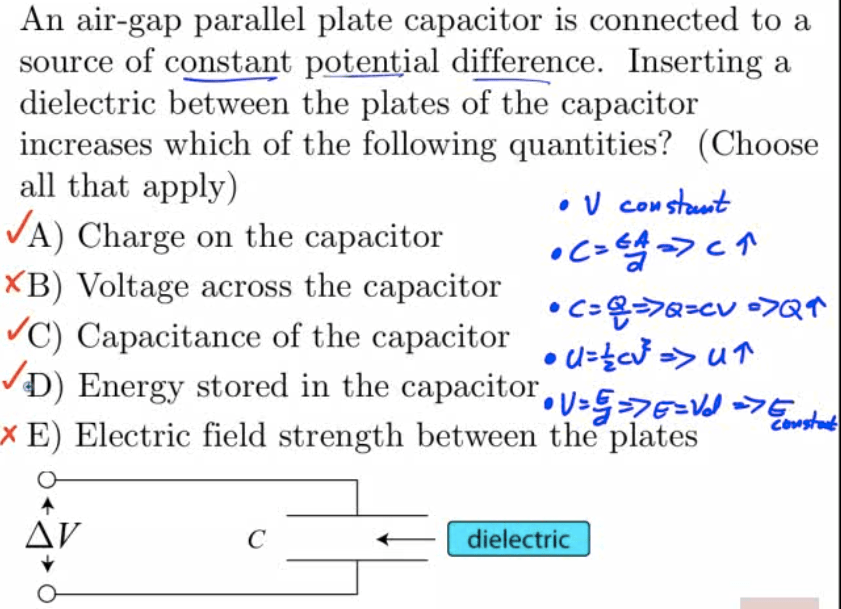# What is a Capacitor?

• A capacitor is an electric device used to store electrical energy

• Two conducting plates

• Insulating material between (dielectric)

• Place opposite charges on each plate

• Develop a potential difference across the plates

• Energy is stored in the electric field between the plates# Capacitance

• Capacitance (C) is the ratio of the charge separated on the plates of a capacitor to the potential difference between the plates

• Units of capacitance are coulombs/volt, or farads (F)

• A farad is a very large amount of capacitance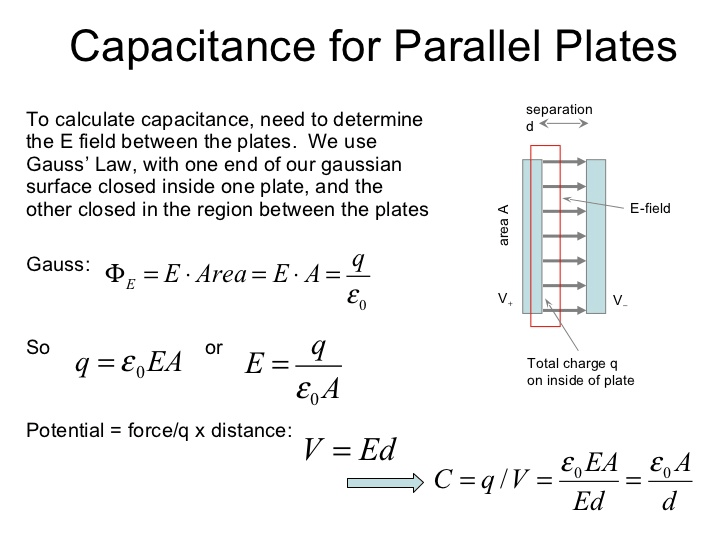# Calculating Capacitance

1. Assume a charge of +Q and -Q on each conductor

2. Find the electric field between the conductors (Gauss's Law)

3.4.# Example 1: Parallel Plates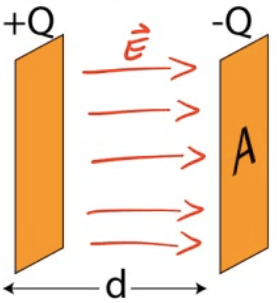• Determine the capacitance between identical parallel plates of area A separated by a distance d
1. Assume +Q and -Q

2.3.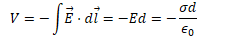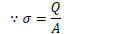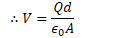4.# Example 2: Cylindrical Capacitor

•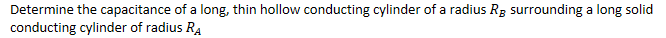1. Assume +Q and -Q
1.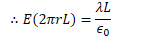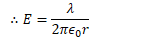2.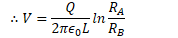3.# Example 3: Spherical Capacitor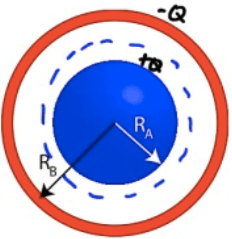•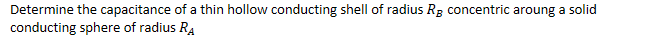1. Assume +Q and -Q
1.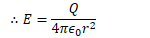2.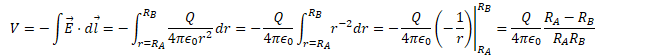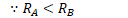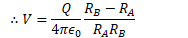3.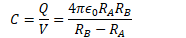# Energy Stored in a Capacitor

• Work is done charging a capacitor, allowing the capacitor to store energy.

••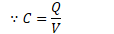•••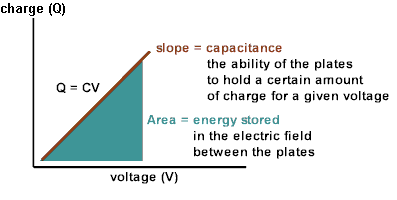# Field Energy Density

•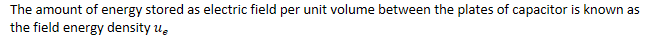•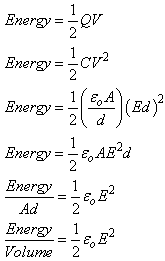# Dielectrics

• Insulating Materials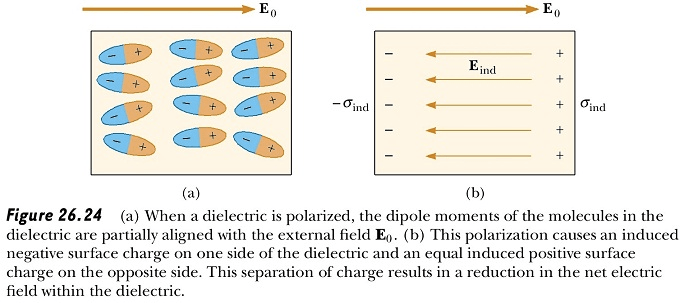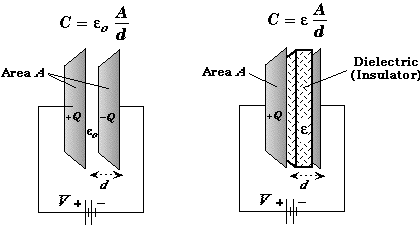# Dielectric Constant (κ)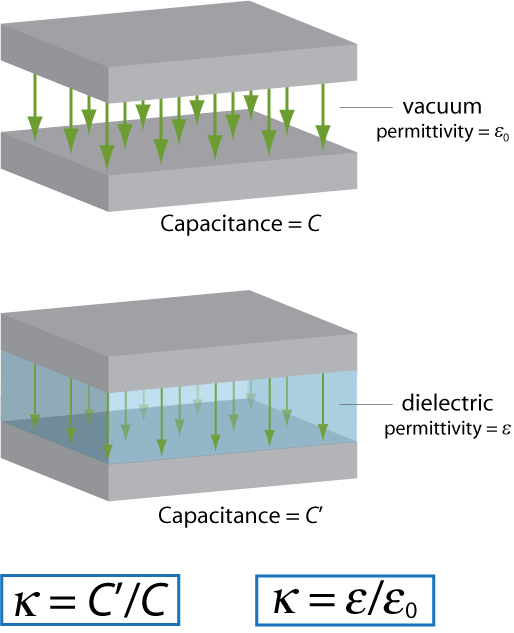# Example 4: Capacitors in Series• Determine the equivalent capacitance of two capacitors in series

••# Example 5: Capacitors in Parallel• Determine the equivalent capacitance of two capacitors in parallel

•# Example 6: Capacitance

• A capacitor stores 3 microcoulombs of charge with a potential difference of 1.5 volts across the plates. What is its capacitance? How much energy is stored in the capacitor?

••# Example 7: Charge on a Capacitor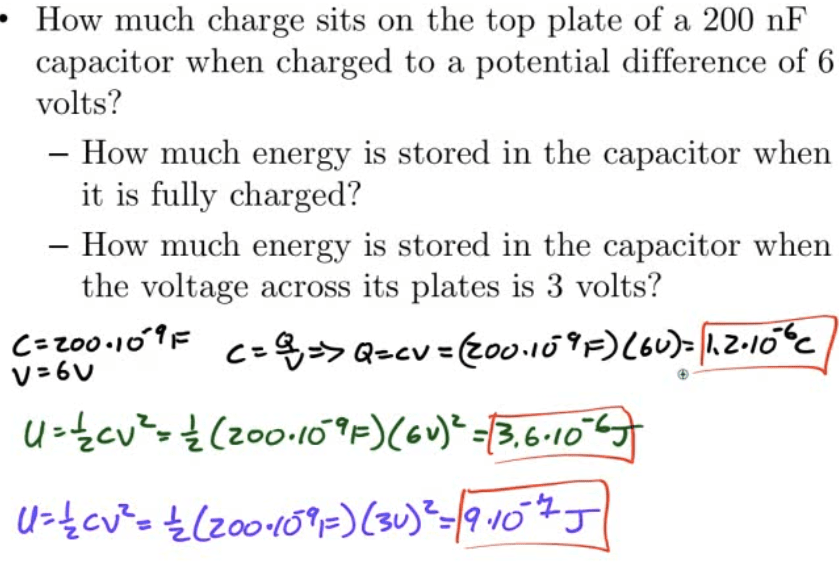# Example 8: Designing a Capacitor# Example 9: Calculating Capacitance# Example 10: Two Conducting Spheres# Example 11: Inserting a Dielectric